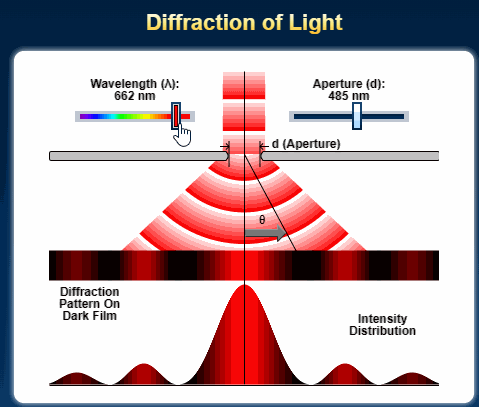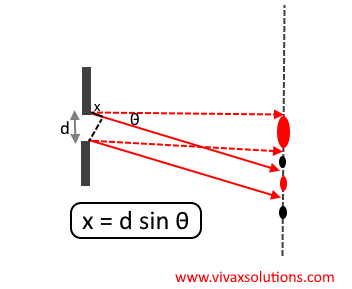### Single Slit Diffraction for A Level PhysicsCredit: Olympus

The diffraction of a beam of light through a single slit is given by the following equation.

x = d sin θ, where x is the path difference and θ is the deviation of the path as an angle. d is the width of the slit or aperture.Single slit diffraction

The greater the angle of diffraction, the greater the value of x.

If x is a multiple of λ, the wavelength, bright spots are visible on the screen. If x is a multiple of 1/2 λ, however, the spots become dark; at those points, constructive and destructive interference take place respectively.

The spot spread on the screen with an equal gap between them.

The values of x for the bright spots can be λ, 2λ, 3λ and so on. For the dark spots, the are 1/2 λ, 3/2 λ, 5/2 λ and so on.

The intensity of the spots, however, goes down with the distance from the central spot, which is twice as wide as other spots.

From the above equation,
sin θ = λ/d

Based on the above formula, the greater the width of aperture, the smaller the angle of diffraction. On the other hand, the smaller the width of the aperture, the greater the diffraction.

For the first bright spot on the screen, x = λ
sin t = λ / d
When diffraction is maximum in theory, sin t = 1
1 = λ / d
d = λ
When diffraction is maximum, the width of the aperture must be equal to the wavelength of the light.